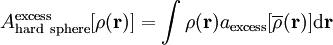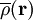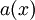# Tarazona's weighted density approximation

(diff) ← Older revision | Latest revision (diff) | Newer revision → (diff)$A_{\mathrm {hard~sphere}}^{\mathrm {excess}} [\rho ({\mathbf r})] = \int \rho ({\mathbf r}) a_{\mathrm {excess}} [\overline\rho ({\mathbf r})]{\mathrm d}{\mathbf r}$,
where$\overline\rho ({\mathbf r})$ is an average of the density distribution and where A is the Helmholtz energy function. The function$a(x)$ should vanish at low values of its argument (so that the excess vanishes and one is left with the ideal case), and diverge at some saturation density corresponding to complete packing.